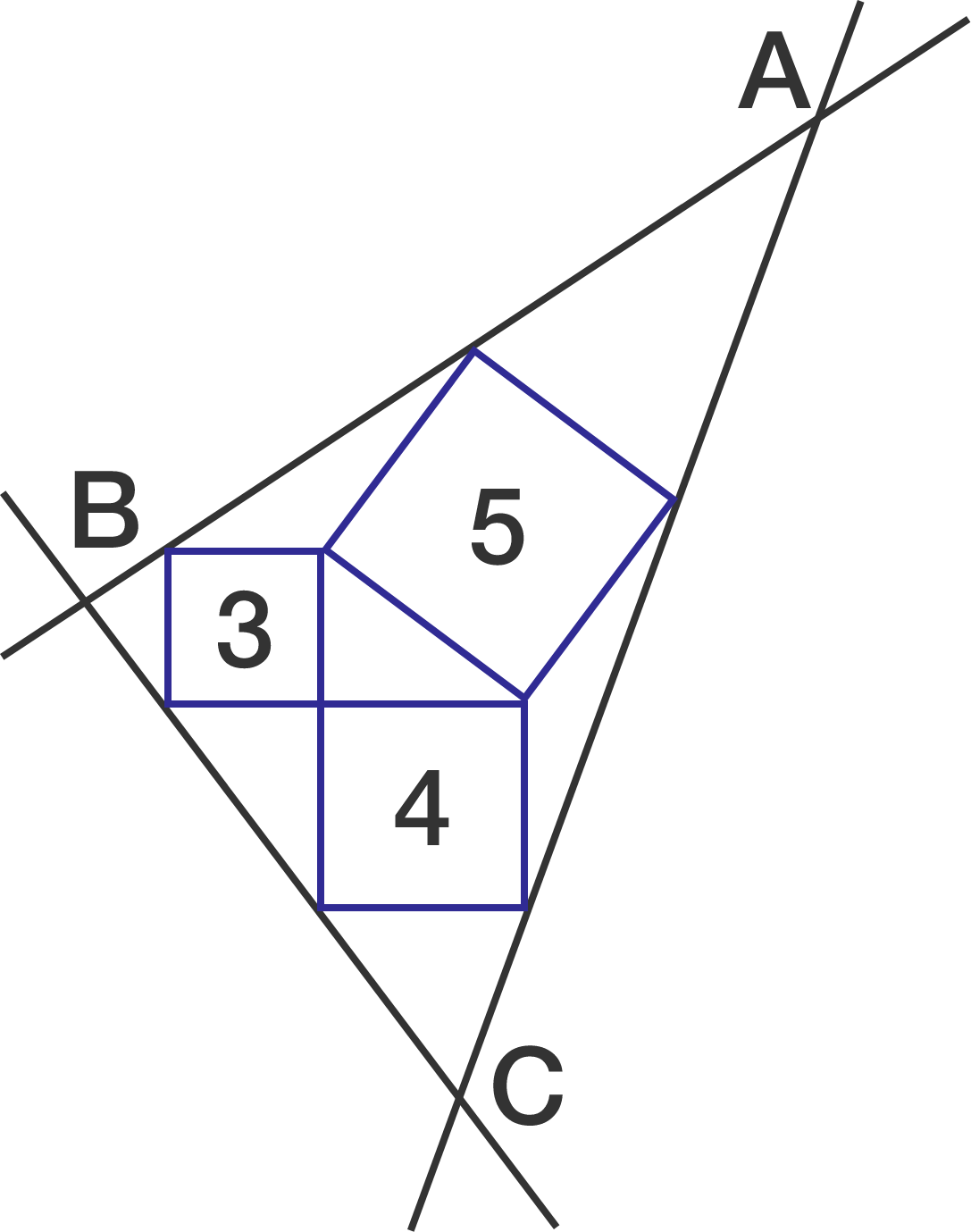# Is it a Right Triangle?

Geometry Level 3A $3-4-5$ right triangle has squares drawn outward on each of its sides. Lines are drawn through the outer corners of these squares, as shown. These lines form a triangle $ABC$.

Is $\Delta ABC$ a right-angled triangle?

×